Lecture 10

# Light Sources

• Point

#### ·       Infinitely Distant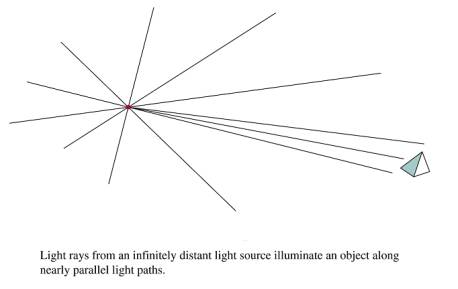• Radial Intensity Attenuation – (1/d2)

#### ·       Problems: Point Source with 1/d2 attenuation does not always produce realistic results.

##### ·       Produces too much intensity variation for near objects and not enough for distant.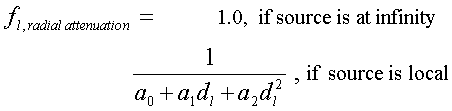#### ·       Directional Light Sources and Spotlight effects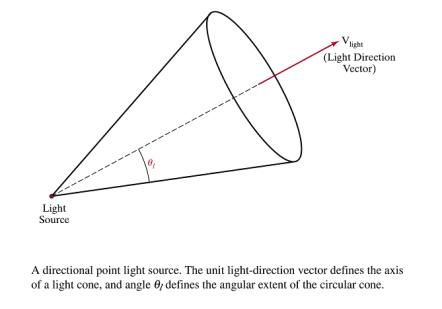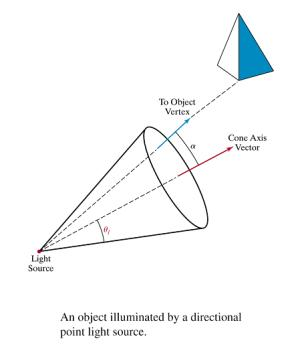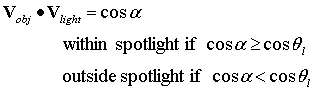#### Angular Intensity Attenuation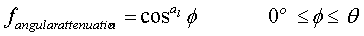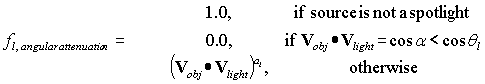# Simple Illumination Model

Surfaces in real world environments receive light in 3 ways:

1.     Directly from existing light sources such as the sun or a lit candle

2.     Light that passes and refracts through transparent objects such as water or a glass vase

3.     Light reflected, bounced, or diffused from other exisiting surfaces in the environment

### Local Illumination

·       Material Models

• Diffuse illumination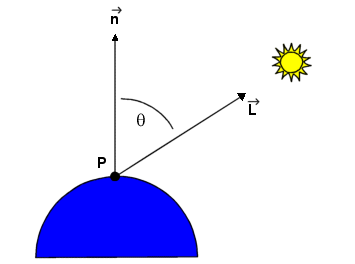Lambert's cosine law of reflection as shown in the above diagram:

1.     n; a normal vector to the surface to be illuminated.

2.     L, a vector from the surface position that points towards the light source.

3.     Il, an intensity for a point light source.

4.     kd , a diffuse reflection constant.

Equation gives the brightness of a surface point in terms of the brightness of a light source and its orientation relative to the surface normal vector, n,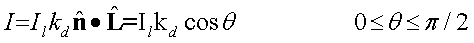·       I is the reflected intensity

Measures how bright the surface is at that point.

·       Surface brightness varies as a function of the angle between n and L

When n and L coincide, the light source is directly overhead.

·       I is at a maximum and cosq = 1.

As the angle increases to 90o, the cosine decreases the intensity to 0.

·       All the quantities in the equation are normalized between 0 and 1.

·       I is converted into frame buffer intensity values by multiplying by the number of shades available.

·       With 28 = 256 possible shades, we have 1 * 255, the brightest frame buffer intensity.

·       For n and L at an angle of 45 o, I = cos 45 o * 256 = 181.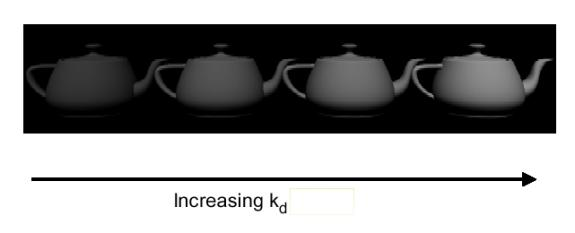• An image rendered with a Lambertian shader exhibits a dull, matte finish.
• It appears as if it has been viewed by a coal miner with a lantern attached to his helmet.
• In reality, an object is not only subjected to direct illumination from the primary light source Il , but secondary scattered light from all remaining surfaces.

• Ambient illumination

·        Simple illuminated model is unable to directly accommodate all scattered light

·        It is grouped together as independent intensity, Ia.

·        The formula becomes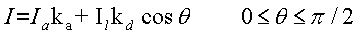·        Iaka is the ambient illumination term, taking into account the additional environmental illumination, Ia, and the ability of the object to absorb it, ka.

·        Below Figure: Only ambient illumination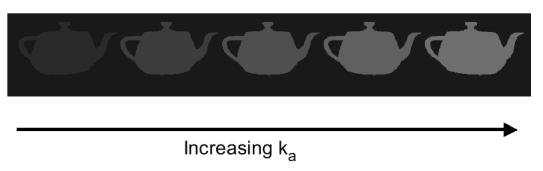• Below Figure: Diffuse + ambient illumination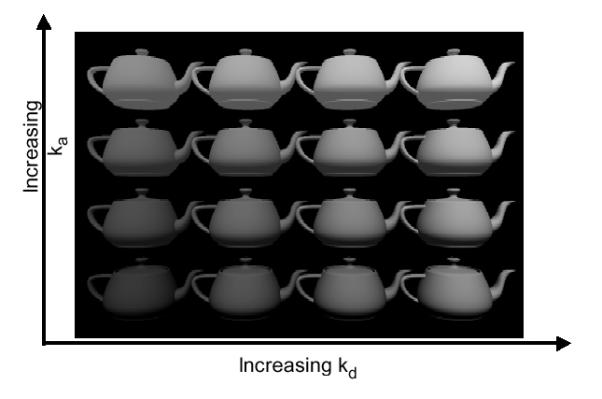• Illumination decreases from the light source by I/d2.
• Objects at a greater distance from the light source receive less illumination and appear darker.
• Above equation is distance independent.
• Dividing the Lambertian term by d2 would seem to get the physics right, but it makes the intensity vary sharply over short distance.
• Modified distance dependence is employed, giving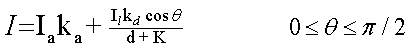·        d is the distance from the light source to the object

• Specular Highlights – (Phong Reflection Model)

·        Regions of significant brightness, exhibited as spots or bands, characterize objects that specularly reflect light.

·        Specular highlights originate from smooth, sometimes mirrorlike surfaces

·        Fresnel equation is used to simulate this effect.

·        The Fresnel equation states that for a perfectly reflecting surface the angle of incidence equals the angle of reflection.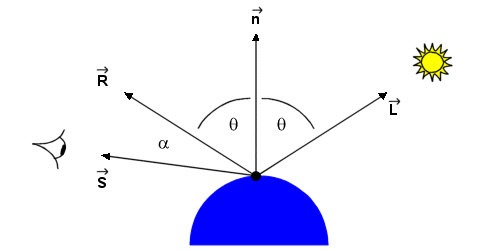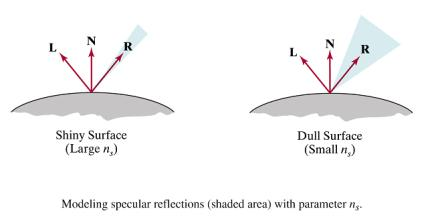·        Most objects are not perfect mirrors.

o      some angular scattering of light.

o      If the viewer increases the angle (a ) between himself, the line of sight vector (S), and the reflectance vector (R), the bright spot gradually disappears.

o      Smooth surfaces scatter light less then rough surfaces.

o      This produces more localized highlights.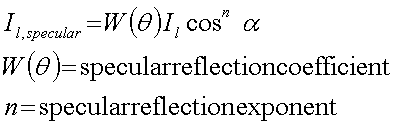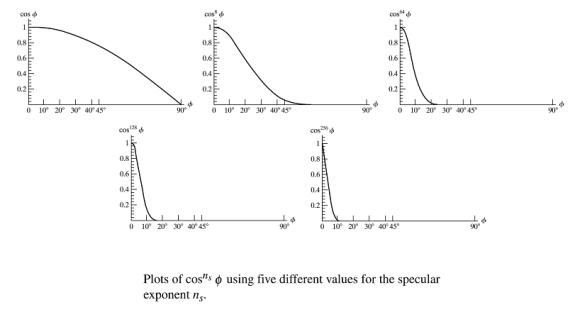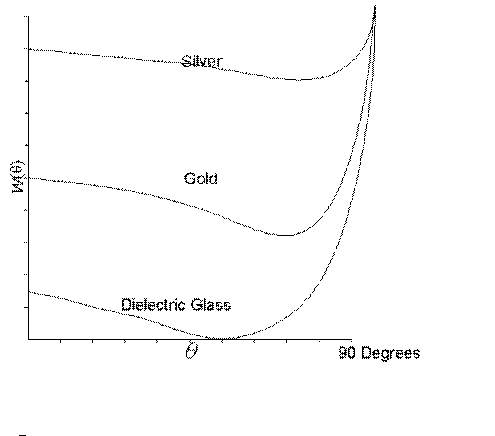o      Building this effect into the lighting model gives·        Specular reflectance term possesses a specular reflectance constant, ks.

·        The cosine term is raised to the nth power.

o      Small values of n (e.g. 5) distribute the specular highlights, characteristic of glossy paper.

o      High values of n (e.g. 50) are characteristic of metals.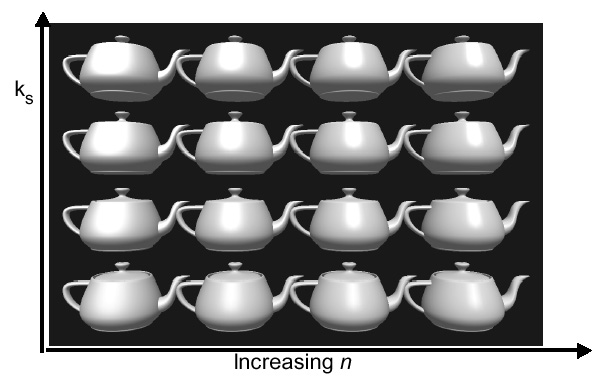1.     Simple Illumination Model

• Deficiencies
• point light source
• no interaction between objects
• ad hoc, not based on model of light propagation
• Benefits
• fast
• acceptable results
• hardware support

## How to Implement

Use the following formula for diffuse + ambient illumination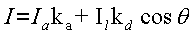0 £ q £ p/2

1.     Determine polygon’s surface normal

2.     Calculate cosine of angle between surface normal and illumination vector

3.     Scale to frame buffer values between 0 and 255

1.     Determine polygon’s surface normal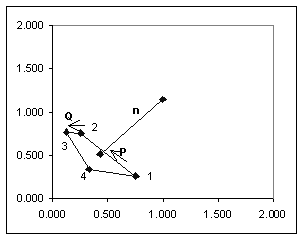i.     Begin with polygon vertices

 x y z 1 0.750 0.250 0.000 2 0.250 0.750 0.000 3 0.125 0.750 0.217 4 0.375 0.250 0.650

ii.     Create vectors P and Q

P = [ P2 – P1] = [ Px  Py  Pz ] = [ x2 – x1  y2 – y1  z2 – z1 ]

= [ -0.500     0.500  0.000 ]

Q = [ P3 – P2] = [ Qx  Qy  Qz ] = [ x2 – x2  y3 – y2  z3 – z2 ]

= [ -0.125      0.000  0.217 ]

iii.     Determine length of P and Q

|P| = sqrt( Px2 + Py2 + Pz2 ) = 0.7071

|Q| = sqrt( Qx2 + Qy2 + Qz2 ) = 0.2500

iv.     Normalize P and Q

P(norm) = P / |P| =  [ Px / |P|  Py / |P|   Pz / |P|  ]

= [ -0.7071    0.7071          0.0000 ]

Q(norm) = Q / |Q| = [ Qx / |Q|  Qy / |Q|   Qz / |Q|  ]

= [ -0.5000    0.0000          0.8660 ]

v.     Calculate normal vector using cross product:

 n = PxQ nx = PyQz - PzQy ny = PzQx -PxQz nz = PxQy - PyQx

n = [nx           nx        nx] = [0.1083             0.1083            0.0625]

vi.     Normalize n

 |n| = 0.165359 n(Norm) = [0.6547 0.6547 0.3780 ]

vii.     Create a light vector

·       Select a point on the polygon surface and position for the light source

o      A polygon vertex may be selected or a position inside the polygon.

o      Here we select a position inside the polygon by calculating the midpoint between the 1st and 3rd vertices

(xmid, ymid, zmid) =  ( (x1 + x3)/2 , (y1 + y3)/2 , (z1 + z3)/2 )

 = (0.438 0.5 0.108)

o      A position for the light source is give as

 x y z 10 10 -5

Note: If observer is in –z direction than z value of light is also –z.

·       Compute light vector L

 Lx Ly Lz Light Vector (L) 9.563 9.500 -5.108 |L| 14.415 L(Norm) 0.66338 0.65905 -0.35438

2.     Calculate cosine of angle between surface normal and illumination vector

i.     Use dot product formula:

 nlL = (nxLx+nyLy+nzLz)=|n||L|cosq

Since n and L are already normalized the formula reduces to

 nlL = (nxLx+nyLy+nzLz) = cosq = 0.7318

3.     Scale to frame buffer values between 0 and 255

0.7318 * 255 = int(186.6) = 186

# Polygon Rendering Methods

Shades each polygon of an object based on the angle between the polygon's surface normal and the direction of the light source.

Disadvantage - it gives low-polygon models a faceted look. Sometimes this look can be advantageous though, such as in modeling boxy objects. Artists sometimes use flat shading to look at the polygons of a solid model they are creating.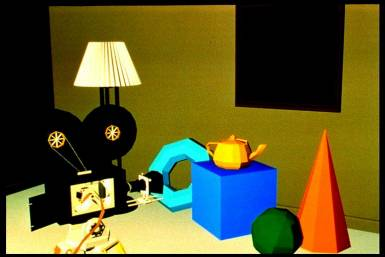Gouraud shading (Henri Gouraud, 1971) is used to achieve smooth lighting on low-polygon surfaces using the Lambertian diffuse lighting model.

1.     Calculates the surface normals for the polygons.

2.     Normals are then averaged for all the polygons that meet at each vertex to produce a vertex normal.

3.     Lighting computations are then performed to produce intensities at vertices.

4.     These intensities are interpolated along the edges of the polygons.

5.     The polygon is filled by lines drawn across it that interpolate between the previously calculated edge intensities.

Advantage - Gouraud shading is superior to flat shading which requires significantly less processing than Gouraud, but gives low-polygon models a sharp, faceted look.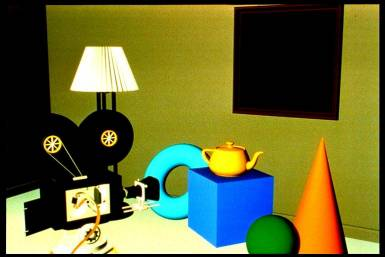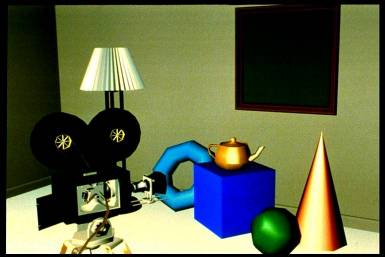#### Phong Shading (Phong Interpolation Model)

·        An improved version of Gouraud shading that provides a better approximation to the Phong shading model.

·        Main problem with Gouraud shading - when a specular highlight occurs near the center of a large triangle, it will usually be missed entirely. Phong shading fixes the problem.

1.     Calculate the surface normals at the vertices of polygons in a 3D computer model.

2.     Normals are then averaged for all the polygons that meet at each vertex.

3.     These normals are interpolated along the edges of the polygons.

4.     Lighting computations are then performed to produce intensities at positions along scanlines.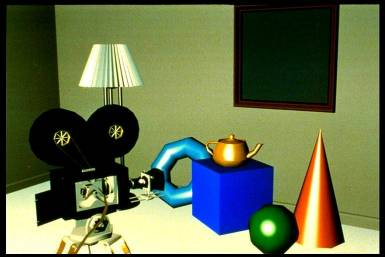# OpenGL Lighting

## Step 1: Enable Lighting

`gl.glEnable(GL.GL_LIGHTING);`

`gl.glEnable(GL.GL_LIGHT0);`

`          `

```float [] lightColor = {1.0f, 1.0f, 1.0f, 1.0f}; ```

```gl.glLightfv(GL.GL_LIGHT0, GL.GL_DIFFUSE, lightColor);```

` `

## Step 2: Position the Light Source

float [] lightPosition = {5, 5, 5, 1};

gl.glLightfv(GL.GL_LIGHT0, GL.GL_POSITION, lightPosition);

## Step 3: Setup Material Properties

`gl.glEnable(GL.GL_COLOR_MATERIAL);`

```gl.glColorMaterial(GL.GL_FRONT_AND_BACK, GL.GL_DIFFUSE);    ```

#### Sample Program: Illuminated Cube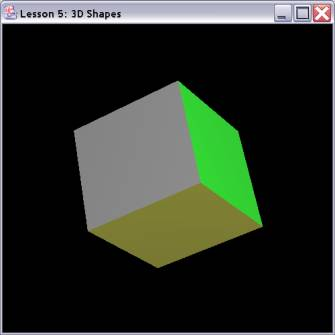import java.awt.*;

import java.awt.event.*;

import net.java.games.jogl.*;

public class CubeLight

{

static Animator animator = null;

static class Renderer

implements GLEventListener,

KeyListener

{

public void display(GLDrawable gLDrawable)

{

final GL gl = gLDrawable.getGL();

gl.glClear(GL.GL_COLOR_BUFFER_BIT | GL.GL_DEPTH_BUFFER_BIT);

gl.glTranslatef(0.0f, 0.0f, -15.0f);

gl.glColor3f(0.0f,1.0f,0.0f);

gl.glNormal3f(0.0f, 1.0f, 0.0f);

gl.glVertex3f( 1.0f, 1.0f,-1.0f);

gl.glVertex3f(-1.0f, 1.0f,-1.0f);

gl.glVertex3f(-1.0f, 1.0f, 1.0f);

gl.glVertex3f( 1.0f, 1.0f, 1.0f);

gl.glColor3f(1.0f,0.5f,0.0f);

gl.glNormal3f(0.0f, -1.0f, 0.0f);

gl.glVertex3f( 1.0f,-1.0f, 1.0f);

gl.glVertex3f(-1.0f,-1.0f, 1.0f);

gl.glVertex3f(-1.0f,-1.0f,-1.0f);

gl.glVertex3f( 1.0f,-1.0f,-1.0f);

gl.glColor3f(1.0f,0.0f,0.0f);

gl.glNormal3f(0.0f, 0.0f, 1.0f);

gl.glVertex3f( 1.0f, 1.0f, 1.0f);

gl.glVertex3f(-1.0f, 1.0f, 1.0f);

gl.glVertex3f(-1.0f,-1.0f, 1.0f);

gl.glVertex3f( 1.0f,-1.0f, 1.0f);

gl.glColor3f(1.0f,1.0f,0.0f);

gl.glNormal3f(0.0f, 0.0f, -1.0f);

gl.glVertex3f( 1.0f,-1.0f,-1.0f);

gl.glVertex3f(-1.0f,-1.0f,-1.0f);

gl.glVertex3f(-1.0f, 1.0f,-1.0f);

gl.glVertex3f( 1.0f, 1.0f,-1.0f);

gl.glColor3f(0.0f,0.0f,1.0f);

gl.glNormal3f(-1.0f, 0.0f, 0.0f);

gl.glVertex3f(-1.0f, 1.0f, 1.0f);

gl.glVertex3f(-1.0f, 1.0f,-1.0f);

gl.glVertex3f(-1.0f,-1.0f,-1.0f);

gl.glVertex3f(-1.0f,-1.0f, 1.0f);

gl.glColor3f(0.5f,0.5f,0.5f);

gl.glNormal3f(1.0f, 0.0f, 0.0f);

gl.glVertex3f( 1.0f, 1.0f,-1.0f);

gl.glVertex3f( 1.0f, 1.0f, 1.0f);

gl.glVertex3f( 1.0f,-1.0f, 1.0f);

gl.glVertex3f( 1.0f,-1.0f,-1.0f);

gl.glEnd();

gl.glFlush();

}

public void displayChanged(GLDrawable gLDrawable, boolean modeChanged, boolean deviceChanged)

{

}

public void init(GLDrawable gLDrawable)

{

final GL gl = gLDrawable.getGL();

final GLU glu = gLDrawable.getGLU();

gl.glClearColor(0.0f, 0.0f, 0.0f, 0.5f);    // Black Background

gl.glClearDepth(1.0f);                      // Depth Buffer Setup

gl.glEnable(GL.GL_DEPTH_TEST);      // Enables Depth Testing

gl.glDepthFunc(GL.GL_LEQUAL); // The Type Of Depth Testing To Do

gl.glMatrixMode(GL.GL_PROJECTION);

gl.glTranslatef(0.0f, 0.0f, 0.0f);

glu.gluPerspective(20.0f, 1.0, 6.0, 20.0);

float [] lightPosition = {5, 5, 5, 1};

gl.glLightfv(GL.GL_LIGHT0, GL.GL_POSITION, lightPosition);

gl.glMatrixMode(GL.GL_MODELVIEW);

gl.glEnable(GL.GL_LIGHTING);

gl.glEnable(GL.GL_LIGHT0);

float [] lightColor = {1.0f, 1.0f, 1.0f, 1.0f};

gl.glLightfv(GL.GL_LIGHT0, GL.GL_DIFFUSE, lightColor);

gl.glEnable(GL.GL_COLOR_MATERIAL);

gl.glColorMaterial(GL.GL_FRONT_AND_BACK, GL.GL_DIFFUSE);

}

public void reshape(GLDrawable gLDrawable, int x, int y, int width, int height)

{

}

public void keyPressed(KeyEvent e)

{

if (e.getKeyCode() == KeyEvent.VK_ESCAPE)

{

animator.stop();

System.exit(0);

}

}

public void keyReleased(KeyEvent e) {}

public void keyTyped(KeyEvent e) {}

}

public static void main(String[] args)

{

Frame frame = new Frame("Lesson 5: 3D Shapes");

GLCanvas canvas = GLDrawableFactory.getFactory().createGLCanvas(new GLCapabilities());

frame.setSize(500, 500);

animator = new Animator(canvas);

{

public void windowClosing(WindowEvent e)

{

animator.stop();

System.exit(0);

}

});

frame.show();

animator.start();

canvas.requestFocus();

}

}